# Flipping Coins in Quantum Computing

Probability. You likely remember being taught about it in school through John having an absurd amount of watermelons. Outside of the scope of fruits, this branch of mathematics is actually an integral part of the foundations of many emerging technologies, especially quantum computing.

Learning how to predict the probability of things is one of the most important progressions of the human species to date. In quantum computing, knowing how to make predictions is integral to its usage.

John has a box of 20 watermelons. He draws a dot on 2 two of them. He gives this box to Sally to pick one with her eyes closed. What is the probability that Sally will pick a watermelon with a dot on it? The old “why on earth does this person have 20 watermelons” question. A question of probability. Let’s take a look at making these predictions, we must first explore how we find the values we will be measuring the probability of.

But before we explore the wondrous world of prediction and chance, let’s take a look at this quote that describes my fascination with this topic from theoretical computer scientist, Dr. Scott Aaronson.

Quantum mechanics is a beautiful generalization of the laws of probability. - Dr. Scott Aaronson

# Measuring Units

## Bits

Classical computers run in a binary, meaning the instructions for operation performed is made up of a specific set of ones and zeros. A bit is the unit of information for these two possible values.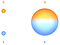Left: Bit Right: Qubit

## Qubits

Qubits, however, hold the probability of the qubits being a one or a zero. This probability is called a quantum superposition state.

However, when we measure the value of the qubit, we aren’t given the probability, a one or zero is revealed which is determined by the probabilities of superposition.

# Superposition

Superposition is a qubit’s ability to be in multiple states at once until it’s measured.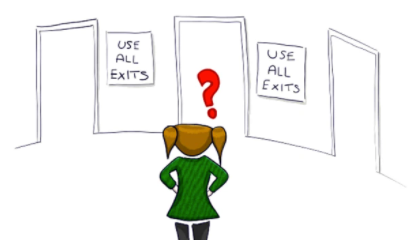Source: Matilda’s Lab

To illustrate this, suppose you spin a coin on your counter. While the coin is spinning, it’s both heads and tails, or perhaps it’s leaning to one side at a minuscule level, but when it falls, it’s either heads or tails. This scenario describes the scenario of measuring a qubit.

Hidden in the definition above, you likely noticed the “until it’s measured”, this is crucial because once it’s measured, the superposition state is destroyed as the value becomes a one or a zero, this is known as the collapsing of a wave function. Superposition also allows us to draw the linear combination of a state. For example, the state 0.3∣0⟩+0.7∣1⟩, is a superposition of ∣0⟩ and |1⟩.

## Wave-particle Duality

What gives rise to quantum computing is wave-particle duality. This is a concept in quantum mechanics which states that every quantum entity or particle can be described as either a wave or a particle.

## Entanglement

Another phenomenon found in the realm of quantum mechanics is quantum entanglement. This describes how when two quantum systems encounter each other, the two become entangled, meaning each carries information about the other no matter what their proximities are to each other. This property demonstrates that entangled qubits cannot be described individually. Manipulating how these electrons encounter is what allows us to accomplish our desired outcome.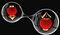Source: Fine Art America

In the picture above we see two entangled electrons, let’s call them x and y. If x is on the opposite side of the earth to y, it can control the movements of the electron it’s entangled with. If you analyze x and measure that it has spin up, then you know in an instant that electron y is spin down. The property of Electron spins can be thought of as how the earth spins on its own axis every twenty-four hours. When an electron spins clockwise on its axis, it is described as a spin up, when an electron spins counterclockwise, it’s described as a spin down.

## Amplitude

Let’s circle back to the watermelons. In the classical laws of physics, it would be impossible for the probability of Sally picking an underripe watermelon to be -3/20. But in terms of quantum physics, whether or not an amplitude is positive or negative is what allows quantum computers to be so powerful.

But how do qubits hold their probabilities? The answer lies in the depths of probability amplitudes, which are complex numbers that describe system behaviours. These can be either positive or negative.

## How Amplitudes Function

When a positive and negative amplitude meet, they cancel each other out, making the outcome closer to 0. When a positive amplitude meets another positive amplitude, the probability of this outcome increases. Because of this, we can manipulate the chances of a particular outcome without measuring it.

They are the coefficient for a specific superposition state. For example, in the state 0.2∣0⟩+0.5∣1⟩, the amplitudes are 0.2 and 0.5.

This is how qubit states are expressed:

In this notation, ψ means wave function and the coefficient 𝝰 represents the amplitude. The part to the left of the equal sign is a quantum state and the part on the right of it is the superposition.

# Representing Quantum States

Quantum states are two-dimensional vectors, meaning they can be represented like so:

These vectors can’t be any old vector, there is a requirement for the vectors used which is that the sums of the squares of the amplitudes must be equal to one. This is called the normalization constraint.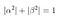Expression (A and B are amplitudes)

For instance, for the state 0.6 |0⟩+0.8∣1⟩ the sum of the squares of the amplitudes is 0.⁶²+0.⁸², which is 0.36+0.64 = 10.36+0.64=1, and therefore satisfying our normalization constraint.

Here, ∣0⟩ and |1⟩ are orthonormal vectors because the two vectors are perpendicular to each other. This vector satisfies the normalization constraint and is thus a normalized vector.

## Computational Basis States

When thinking about qubits and bits, they aren’t fully different. There are two particular quantum states that are in correspondence with the binary. How these states are represented is through computational basis states. These are any of the two quantum states that a qubit can be in physically. ∣ and ⟩ are ket notations while its entirety is a ket.

# Vector Spaces

As mentioned before, a quantum state is a two-dimensional vector space, however, its entry state can actually be a complex number! But as for their computational basis states, they must have real entries. In this example, the numbers shown are real, but not inherently.

Allow me to explain. In this representation, there are only real numbers. However, the “i”s represent imaginary numbers. This means that it is a complex number which can also be written as a real number multiplied by the imaginary unit.

This is defined by its property: i² = -1

Let’s take 4i for example, this is an imaginary number and its square would be -16.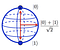Source: Towards data science

# Representing Qubits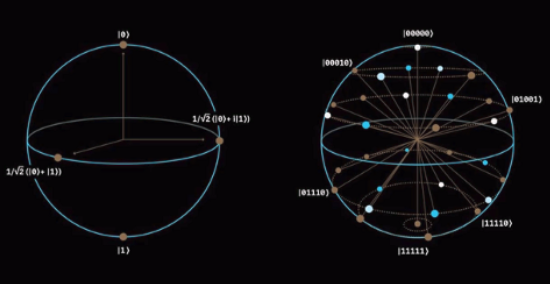Source: IBM Research

Bloch Sphere (left):

• Standard way of representing two-bit states
• Qubits are represented as points on a unit sphere

Qsphere (right):

• Can represent multi-qubit states as well as single-bit states
• For example, it could represent a 4-qubit state

# Quantum Logic Gates

So far, we have discussed understanding quantum states and how we represent them, but how do we use them? The answer is quantum logic gates. These are what allow us to use quantum states to compute! Quantum logic gates, like classical logic gates, are what allow us to manipulate data. In this case, we are manipulating a qubit or group of qubit’s quantum state to allow for the desired function. The functions of the gates themselves are beyond the scope of this article, but here is a great article explaining them.

## Quantum Computing in the World Around Us

Before I go, I want to touch on why we should care about John’s fruit and electrons being entangled. The answer is that quantum computing is evolving, and fast. It’s the future. Although they won’t be replacing classical computers any time soon, they are the key to unlocking new possibilities in computing. It’s already revolutionizing countless fields today and has a multitude of uses in areas such as:

• Accelerated drug testing
• Forecasting weather and climate change
• Stronger cybersecurity
• Cleaner fertilization
• Better batteries
• Optimizing traffic
• Developing drugs
• Financial models

## TL;DR

• A bit represents a logical state with only two possible values in the binary of one and zero whereas a qubit can be in both states simultaneously
• Superposition allows qubits to be coherently in both states 1 and 0 coherently
• A qubits quantum state is a vector of unit-length in a two-dimensional vector space called the state space
• Quantum logic gates are how we use manipulate quantum states
• Quantum computing is the future and understanding it will allow us to use it in many fields to change the way we predict

Hello there, my name’s Laura and I’m a curious 16-year-old hoping to have an impact on the world!

If you would like to follow along with my journey, you can connect with me on LinkedIn and subscribe to my monthly newsletter.

17-year-old Innovator at The Knowledge Society